SNVSBI6 January   2021

PRODUCTION DATA

1. Features
2. Applications
3. Description
4. Revision History
5. Description (continued)
6. Device Comparison Table
7. Pin Configuration and Functions
8. Specifications
9. Detailed Description
1. 9.1 Overview
2. 9.2 Functional Block Diagram
3. 9.3 Feature Description
4. 9.4 Device Functional Modes
10. 10Application and Implementation
1. 10.1 Application Information
2. 10.2 Typical Application
1. 10.2.1 Design Requirements
2. 10.2.2 Detailed Design Procedure
3. 10.2.3 Application Curve
3. 10.3 System Examples
11. 11Power Supply Recommendations
12. 12Layout
13. 13Device and Documentation Support
1. 13.1 Device Support
2. 13.2 Documentation Support
3. 13.3 Receiving Notification of Documentation Updates
4. 13.4 Support Resources
5. 13.5 Trademarks
6. 13.6 Electrostatic Discharge Caution
7. 13.7 Glossary
14. 14Mechanical, Packaging, and Orderable Information

• DSS|12
• DSS|12

#### 10.2.2.8 Efficiency Estimation

The total loss of the boost converter (PTOTAL) can be expressed as the sum of the losses in the device (PIC), MOSFET power losses (PQ), diode power losses (PD), inductor power losses (PL), and the loss in the sense resistor (PRS).

Equation 19.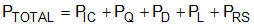PIC can be separated into gate driving loss (PG) and the losses caused by quiescent current (PIQ).

Equation 20.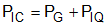Each power loss is approximately calculated as follows:

Equation 21.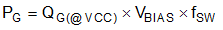Equation 22.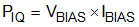IVIN and IVOUT values in each mode can be found in the supply current section of Section 8.5.

PQ can be separated into switching loss (PQ(SW)) and conduction loss (PQ(COND)).

Equation 23.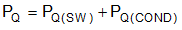Each power loss is approximately calculated as follows:

Equation 24.tR and tF are the rise and fall times of the low-side N-channel MOSFET device. ISUPPLY is the input supply current of the boost converter.

Equation 25.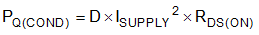RDS(ON) is the on-resistance of the MOSFET and is specified in the MOSFET data sheet. Consider the RDS(ON) increase due to self-heating.

PD can be separated into diode conduction loss (PVF) and reverse recovery loss (PRR).

Equation 26.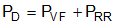Each power loss is approximately calculated as follows:

Equation 27.Equation 28.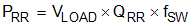QRR is the reverse recovery charge of the diode and is specified in the diode data sheet. Reverse recovery characteristics of the diode strongly affect efficiency, especially when the output voltage is high.

PL is the sum of DCR loss (PDCR) and AC core loss (PAC). DCR is the DC resistance of inductor which is mentioned in the inductor data sheet.

Equation 29.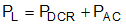Each power loss is approximately calculated as follows:

Equation 30.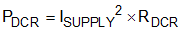Equation 31.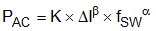Equation 32.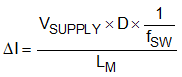∆I is the peak-to-peak inductor current ripple. K, α, and β are core dependent factors which can be provided by the inductor manufacturer.

PRS is calculated as follows:

Equation 33.Efficiency of the power converter can be estimated as follows:

Equation 34.